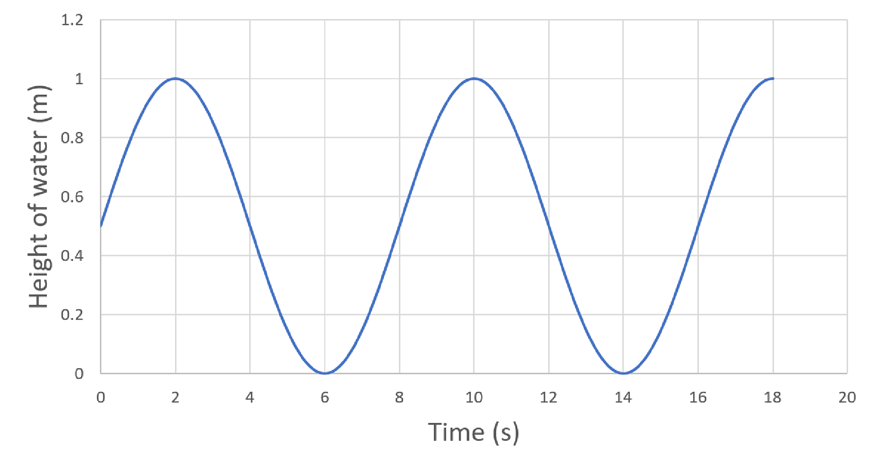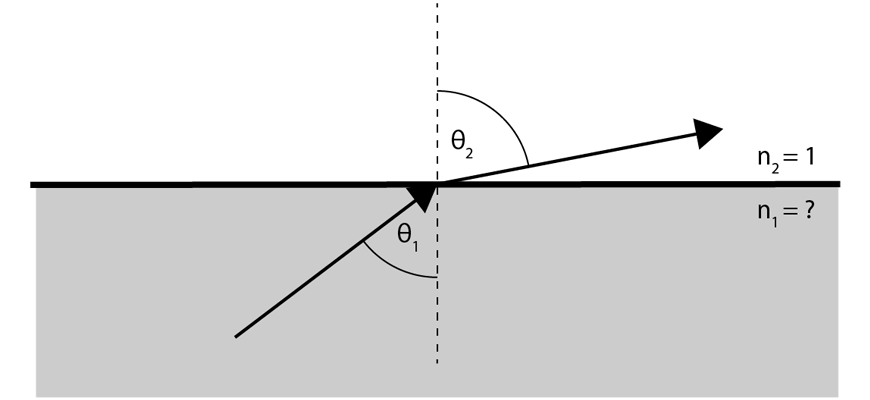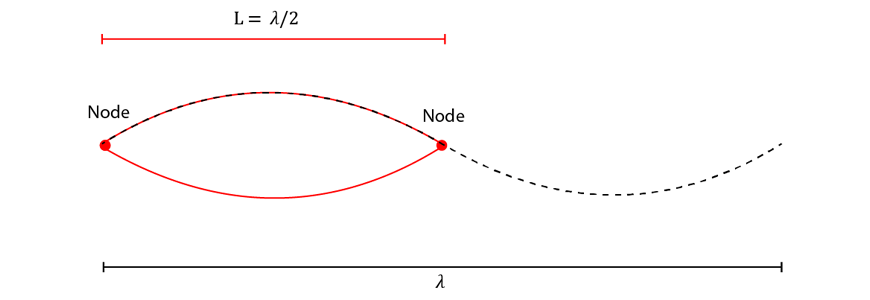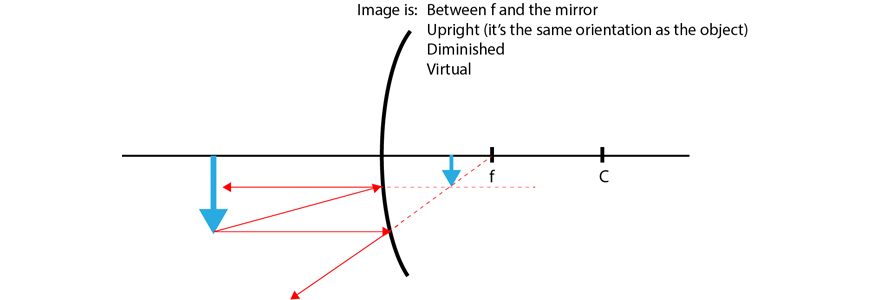# Year 11 Physics Waves and Thermodynamics Practice Questions

## Year 11 Physics: Waves and Thermodynamics Practice

Below are ten questions to test how well you know Waves and Thermodynamics for Year 11 Physics. We’ve put the answers down the bottom of the page, here.

Get the edge for your next Physics Assessment!

### Question 1:

A student records the passage of water waves as they pass by. Using a large stick, the student records the height of the water as a function of time:a) Determine the amplitude of the wave.

The speed of the wave was later determined to be $$6.5\text{ ms}^{-1}$$

b) Determine the period of the wave.

c) Calculate the wavelength of the wave.

### Question 2:

A student records the frequency of an ambulance siren as it drives past them. When stationary, the frequency of the siren is $$1030 \text{ Hz}$$. The speed of sound in air is $$340\text{ ms}^{-1}$$.

a) Calculate the frequency the student records when the ambulance approaches the student at $$15\text{ ms}^{-1}$$

b) Calculate the velocity of the ambulance if the observed frequency is $$1000 \text{ Hz}$$

c) Determine the frequency recorded the instant the ambulance passes the student.

### Question 3:

A student is using measurements of light to determine the distance between them and a nearby lighthouse. At some distance $$r$$, they measure the intensity of light to be $$0.0622\text{ Wm}^{-2}$$. After moving $$50\text{ m}$$ closer, they measure the intensity of light to be $$0.14\text{ Wm}^{-2}$$. Using this information, calculate the original distance $$r$$.

### Question 4:

When two sound waves of similar frequencies are played together, they appear to periodically increase and decrease in volume – this phenomena is known as beating.

a) Explain how the wave properties of superposition and interference give rise to beating.

b) Calculate the beat frequency of two tones played simultaneously: one at $$1040 \text{ Hz}$$, and one at $$1045 \text{ Hz}$$.

c) Determine the underlying pitch heard in case b).

### Question 5:

A student performed an investigation of the refraction of light as it transitions from glass to air, as seen in the below diagram:The student recorded values for the incident angle $$\theta_1$$ and exit angle $$\theta_2$$ below:

 Incident Angle (degrees) Exit Angle (degrees) 10 12 20 24 30 37 40 50 50 67 60 N/A 70 N/A

a) Using the gathered data, estimate the refractive index of the glass.

b) Explain why there are no exit angle measurements for the final two data points.

### Question 6:

Outline the following wave phenomena, giving examples for each:

a) Refraction

b) Dispersion

c) Reflection

d) Diffraction

### Question 7:

A Student is investigating the changes in pitch of a $$1\text{ m}$$ long guitar string, which is fixed at both ends. The student assumes that only the fundamental mode of the guitar string is excited.

a) Using a diagram, show that the relationship between the length of the string $$L$$ and the wavelength of the fundamental mode $$\lambda$$ is $$L = \frac{\lambda}{2}$$.

b) Calculate the frequency of the fundamental mode if the speed of waves in the string is $$430 \text{ ms}^{-1}$$.

c) Calculate the length of string required to produce a tone of $$600 \text{ Hz}$$ (assuming the fundamental mode only is excited).

### Question 8:

An object is placed in-front of a convex spherical mirror as shown below:Using the ray theory of light, determine the location and characteristics of the image that is generated.

### Question 9:

Explain the following concepts in terms of kinetic and internal energy:

a) Temperature.

b) Specific heat capacity.

c) Thermal equilibrium.

d) Thermal conductivity.

### Question 10:

Find the final temperature and heat transferred in the following circumstances. The relevant physical constants of water are:

 Latent heat of fusion (L) $$334,000 \text{ Jkg}^{-1}$$ Heat capacity (c) $$4,184 \text{ Jkg}^{-1}\text{K}^{-1}$$

a) A $$200\text{ g}$$ cup of water at $$0\text{ }^{\circ}\text{C}$$ is placed in a room (bath) of temperature $$25\text{ }^{\circ}\text{C}$$.

b) A $$200\text{ g}$$ cup of ice at $$0\text{ }^{\circ}\text{C}$$ is placed in a room (bath) of temperature $$25\text{ }^{\circ}\text{C}$$.

c) A $$100\text{ g}$$ cup of water at $$10\text{ }^{\circ}\text{C}$$ is mixed with $$400\text{ g}$$ of water at $$27\text{ }^{\circ}\text{C}$$.

## Solutions

Question 1:

a) $$0.5\text{ m}$$

b) $$8\text{ s}$$

c) $$52\text{ m}$$

Question 2:

a) $$1078\text{ Hz}$$

b) $$10.2\text{ ms}^{-1}$$ away from the student.

c) $$1030\text{ Hz}$$ – there is no change.

Question 3

$$150 \text{ m}$$

Question 4

a) Beating arises from two waves superimposing and interfering differently over time, alternating between being in phase (constructive interference, louder sound) and out of phase (destructive interference, softer sound).

b) $$5\text{ Hz}$$

c) $$1042.5\text{ Hz}$$

Question 5

a) $$n_1 = 1.20$$

b) The critical angle for this interface is $$56.4^{\circ}$$. At incident angles larger than this, light does not pass through the interface – it is subject to total internal reflection.

Question 6

a) Refraction – the change in direction of propagation when a wave moves into a medium with a different wave speed. If the wave slows down, the ray bends toward the normal. If the wave speeds up, the ray bends away from the normal.

b) Dispersion – waves of different frequency experience different refractive indices (wave speeds) in the same medium, and hence refract by different amounts when entering this medium. This results in the wave being spread out according to wavelength – like in a rainbow.

c) Reflection – the bouncing of a wave off a barrier like a mirror: the reflected angle is the same as the incident angle (relative to the normal).

d) Diffraction – the bending of a wave around obstacles. More commonly diffraction refers to the expansion of a wavefront through a slit of similar dimensions to the wavelength.

Question 7

a) The standing wave is as follows:b) $$f = 215\text{ Hz}$$

c) $$0.36 \text{ m}$$

Question 8Question 9

a) A measure of the average random kinetic energy of the particles in a system.

b) The amount of energy required to increase the temperature of 1 kg of a material by 1 K.

c) Two objects are in thermal equilibrium when the average random kinetic energy of their constituent particles are equal (i.e. when they have equal temperature).

d) A measure of how easily heat flows through a material, or how easily a material is able to transfer heat through conduction.

Question 10

a) Equilibrium temperature is $$25\text{ }^\circ\text{C}$$. Heat transferred is $$Q = 20,920 \text{ J}$$

b) Equilibrium temperature is $$25\text{ }^\circ\text{C}$$. Heat transferred is $$Q = 87,720 \text{ J}$$

c) Equilibrium temperature is $$23.6\text{ }^\circ\text{C}$$. Heat transferred is $$Q = 5,690 \text{ J}$$

,

#### Related Posts

Platform Slot Gacor CMS Checker